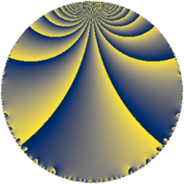# Properties

 Label 43.4.eLevel $43$ Weight $4$ Character orbit 43.e Rep. character $\chi_{43}(4,\cdot)$ Character field $\Q(\zeta_{7})$ Dimension $60$ Newform subspaces $1$ Sturm bound $14$ Trace bound $0$

# Related objects

## Defining parameters

 Level: $$N$$ $$=$$ $$43$$ Weight: $$k$$ $$=$$ $$4$$ Character orbit: $$[\chi]$$ $$=$$ 43.e (of order $$7$$ and degree $$6$$) Character conductor: $$\operatorname{cond}(\chi)$$ $$=$$ $$43$$ Character field: $$\Q(\zeta_{7})$$ Newform subspaces: $$1$$ Sturm bound: $$14$$ Trace bound: $$0$$

## Dimensions

The following table gives the dimensions of various subspaces of $$M_{4}(43, [\chi])$$.

Total New Old
Modular forms 72 72 0
Cusp forms 60 60 0
Eisenstein series 12 12 0

## Trace form

 $$60q - 9q^{2} - 3q^{3} - 31q^{4} - 23q^{5} + 16q^{6} + 96q^{7} + 61q^{8} - 177q^{9} + O(q^{10})$$ $$60q - 9q^{2} - 3q^{3} - 31q^{4} - 23q^{5} + 16q^{6} + 96q^{7} + 61q^{8} - 177q^{9} - 61q^{10} + 83q^{11} + 33q^{12} + 107q^{13} - 299q^{14} + 109q^{15} + 41q^{16} + 181q^{17} - 414q^{18} + 284q^{19} - 363q^{20} - 88q^{21} + 421q^{22} + 231q^{23} - 937q^{24} + 213q^{25} + 139q^{26} - 27q^{27} + 29q^{28} - 367q^{29} + 1244q^{30} - 319q^{31} + 435q^{32} - 2594q^{33} - 583q^{34} - 902q^{35} + 1552q^{36} + 1020q^{37} + 1251q^{38} - 1571q^{39} + 1263q^{40} + 293q^{41} - 1830q^{42} + 1661q^{43} + 6512q^{44} + 1019q^{45} - 2786q^{46} - 287q^{47} - 95q^{48} + 772q^{49} - 282q^{50} + 1524q^{51} - 1511q^{52} - 1505q^{53} - 3489q^{54} - 1735q^{55} - 1237q^{56} + 1055q^{57} + 335q^{58} + 571q^{59} - 101q^{60} - 339q^{61} + 923q^{62} - 702q^{63} - 5163q^{64} + 2463q^{65} + 985q^{66} - 241q^{67} + 2904q^{68} + 2711q^{69} - 7698q^{70} - 2431q^{71} - 4340q^{72} - 2157q^{73} - 1294q^{74} - 242q^{75} - 4272q^{76} - 3962q^{77} - 2860q^{78} + 1092q^{79} + 11618q^{80} + 12060q^{81} + 4023q^{82} - 2664q^{83} + 3334q^{84} - 3446q^{85} + 10055q^{86} + 11874q^{87} + 9957q^{88} - 5811q^{89} - 1612q^{90} - 760q^{91} + 2120q^{92} + 3994q^{93} + 6057q^{94} + 379q^{95} - 2044q^{96} - 5509q^{97} - 9041q^{98} - 2012q^{99} + O(q^{100})$$

## Decomposition of $$S_{4}^{\mathrm{new}}(43, [\chi])$$ into newform subspaces

Label Dim. $$A$$ Field CM Traces $q$-expansion
$$a_2$$ $$a_3$$ $$a_5$$ $$a_7$$
43.4.e.a $$60$$ $$2.537$$ None $$-9$$ $$-3$$ $$-23$$ $$96$$